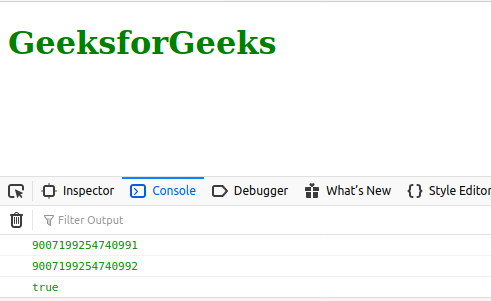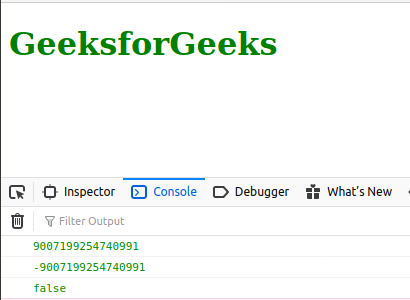# JavaScript Number.MAX_SAFE_INTEGER Constant

Below is the example of the Number.MIN_SAFE_INTEGER Constant.

• Example:

 `<``script` `type``=``"text/javascript"``> ` `    ``document.write(Number.MIN_SAFE_INTEGER);  ` `  `

• Output:

`9007199254740991`

The JavaScript Number.MAX_SAFE_INTEGER is a constant number that represents the minimum safe integer. This constant has a value of (253 – 1). Here safe refers to the ability to represent integers and to compare them.

Example:

`Number.MAX_SAFE_INTEGER + 1 === Number.MAX_SAFE_INTEGER + 2`

The above expression will evaluate to true which is actually mathematically NOT correct.

Syntax:

```Number.MAX_SAFE_INTEGER
```

Return Value: A constant number.

Example 1: Below example illustrates the usage of Number.MAX_SAFE_INTEGER

 ` ` `<``html` `lang``=``"en"``> ` ` `  `<``body``> ` `    ``<``h1` `style``=``"color: green;"``>GeeksforGeeks ` `    ``<``script` `type``=``"text/javascript"``> ` `        ``const a = Number.MAX_SAFE_INTEGER + 1; ` `        ``const b = Number.MAX_SAFE_INTEGER + 2; ` ` `  `        ``console.log(Number.MAX_SAFE_INTEGER); ` `        ``console.log(a); ` `        ``console.log(a === b); ` `    `` ` ` ` ` `  ` `

Output:Example 2: Below example illustrates the usage of the constant Number.MAX_SAFE_INTEGER using Math.pow() function.

 ` ` `<``html` `lang``=``"en"``> ` ` `  `<``body``> ` `    ``<``h1` `style``=``"color: green;"``>GeeksforGeeks ` `    ``<``script` `type``=``"text/javascript"``> ` `        ``const a = Number.MAX_SAFE_INTEGER; ` `        ``const b = -(Math.pow(2, 53) - 1); ` ` `  `        ``console.log(a); ` `        ``console.log(b); ` `        ``console.log(a === b); ` `    `` ` ` ` ` `  ` `

Output:Supported Browsers:

• Chrome
• Internet Explorer (Not Supported)
• Firefox
• Edge
• Safari
• OperaMy Personal Notes arrow_drop_upCheck out this Author's contributed articles.

If you like GeeksforGeeks and would like to contribute, you can also write an article using contribute.geeksforgeeks.org or mail your article to contribute@geeksforgeeks.org. See your article appearing on the GeeksforGeeks main page and help other Geeks.

Please Improve this article if you find anything incorrect by clicking on the "Improve Article" button below.